# Relations And Functions For Class 12

Relations and Functions Class 12 Chapter 1 starts with the revision of general notation of relations and functions. Students have already learned about domain, codomain and range in class 11, along with the various types of specific real-valued functions and the respective graphs. In class 12 Maths Chapter 1, students will learn about different types of relations and functions, the composition of functions, etc., in detail.

## Relations and Functions For Class 12 Concepts

The topics and subtopics covered in relations and Functions for class 12 are:

• Introduction
• Types of Relations
• Types of Functions
• Composition of functions and invertible functions
• Binary operations

Let us discuss the concept of relation and function in detail here.

## Relation

The concept of relation is used in relating two objects or quantities with each other. Suppose two sets are considered. The relationship between them will be established if there is a connection between the elements of two or more non-empty sets.

Mathematically, “a relation R from a set A to a set B is a subset of the cartesian product A × B obtained by describing a relationship between the first element x and the second element y of the ordered pairs in A × B”.

### Types of Relations

A relation R from A to A is also stated as a relation on A, and it can be said that the relation in a set A is a subset of A × A. Thus, the empty set φ and A × A are two extreme relations. Below are the definitions of types of relations:

Empty Relation

If no element of A is related to any element of A, i.e. R = φ ⊂ A × A, then the relation R in a set A is called empty relation.

Universal Relation

If each element of A is related to every element of A, i.e. R = A × A, then the relation R in set A is said to be universal relation.

Both the empty relation and the universal relation are sometimes called trivial relations.

A relation R in a set A is called-

Reflexive- if (a, a) ∈ R, for every a ∈ A,

Symmetric- if (a1, a2) ∈ R implies that (a2, a1) ∈ R , for all a1, a2∈ A,

Transitive- if (a1, a2) ∈ R and (a2, a3) ∈ R  implies that (a1, a3) ∈ R  for all a1, a2, a3 ∈ A.

Equivalence Relation- A relation R in a set A is an equivalence relation if R is reflexive, symmetric and transitive.

## Functions

A function is a relationship which explains that there should be only one output for each input. It is a special kind of relation(a set of ordered pairs) which obeys a rule, i.e. every y-value should be connected to only one y-value.

Mathematically, “a relation f from a set A to a set B is said to be a function if every element of set A has one and only one image in set B”.

In other words, a function f is a relation from a set A to set B such that the domain of f is A and no two distinct ordered pairs in f have the same first element. Also, A and B are two non-empty sets.

### Types of Functions

1. One to one Function: A function f : X → Y is defined to be one-one (or injective), if the images of distinct elements of X under f are distinct, i.e., for every x1 , x2 ∈ X, f(x1 ) = f(x2 ) implies x1 = x2 . Otherwise, f is called many-one.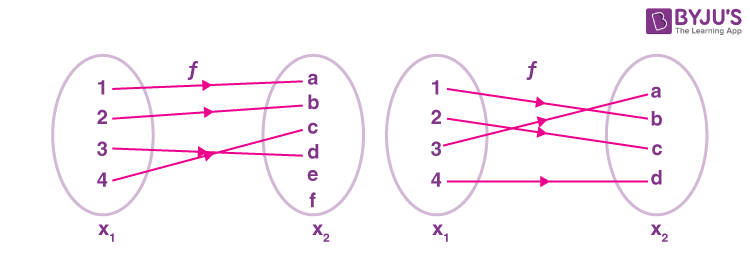One-one function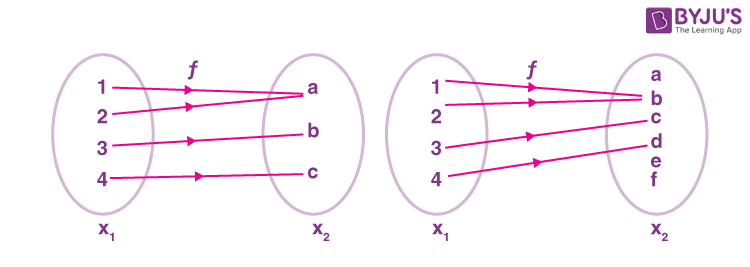Many-one function

2. Onto Function: A function f: X → Y is said to be onto (or surjective), if every element of Y is the image of some element of X under f, i.e., for every y ∈ Y, there exists an element x in X such that f(x) = y.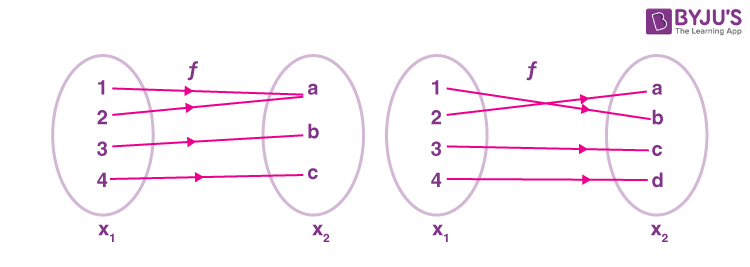Onto function

3. One-one and Onto Function: A function f: X → Y is said to be one-one and onto (or bijective), if f is both one-one and onto.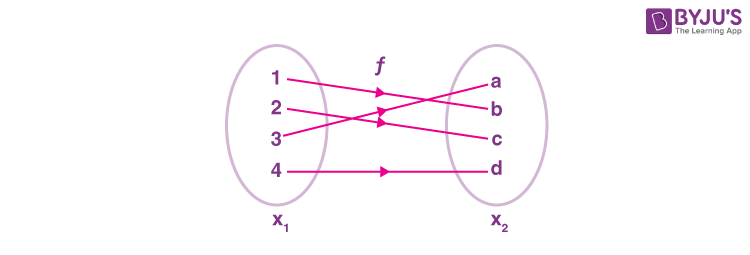One-one and Onto function

### Composition of Functions

Let f: A → B and g: B → C be two functions. Then the composition of f and g, denoted by gof, is defined as the function gof: A → C given by;

gof (x) = g(f (x)), ∀ x ∈ A

### Invertible Functions

A function f : X → Y is defined to be invertible if there exists a function g : Y → X such that gof = IX and fog = IY. The function g is called the inverse of f and is denoted by f–1.

An important note is that, if f is invertible, then f must be one-one and onto and conversely, if f is one-one and onto, then f must be invertible.

### Binary Operations

A binary operation ∗ on a set A is a function ∗ : A × A → A. We denote ∗ (a, b) by a ∗ b.

## Video Lesson on Revision of Relations and Functions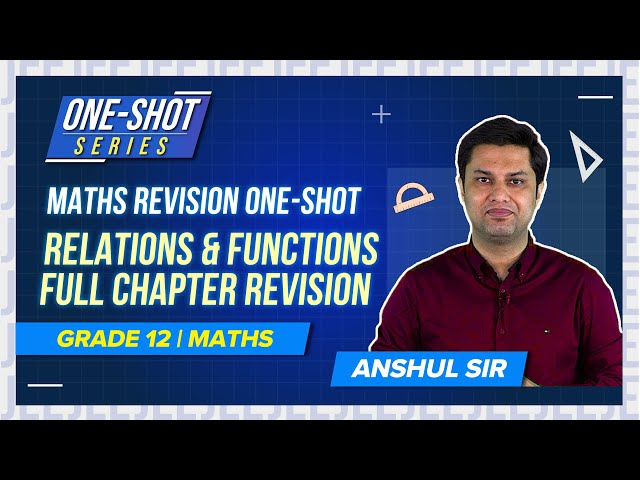## Video Lesson on Paper Analysis

### Relations and Functions Class 12 Maths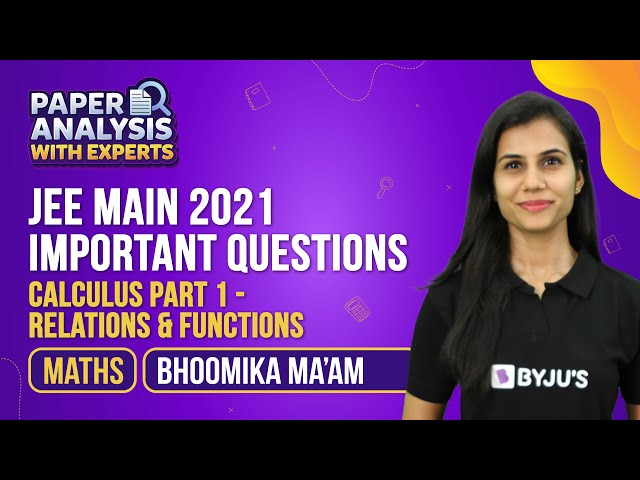## Solved Examples

Example 1: Show that subtraction and division are not binary operations on R.

Solution: N × N → N, given by (a, b) → a – b, is not binary operation, as the image of (2, 5) under ‘–’ is 2 – 5 = – 3 ∉ N.

Similarly, ÷: N × N → N, given by (a, b) → a ÷ b is not a binary operation, as the image of (2, 5) under ÷ is 2 ÷ 5 = 2/5 ∉ N.

Example 2: Let f : {2, 3, 4, 5} → {3, 4, 5, 9} and g : {3, 4, 5, 9} → {7, 11, 15} be functions defined as f(2) = 3, f(3) = 4, f(4) = f(5) = 5 and g (3) = g (4) = 7 and g (5) = g (9) = 11. Find gof.

Solution: From the given, we have:
gof(2) = g (f(2)) = g (3) = 7
gof (3) = g (f(3)) = g (4) = 7
gof(4) = g (f(4)) = g (5) = 11
gof(5) = g (5) = 11

Example 3: Show that the relation R in the set Z of integers given by R = {(a, b) : 2 divides a – b} is an equivalence relation.

Solution: R is reflexive, as 2 divides (a – a) for all a ∈ Z.
Further, if (a, b) ∈ R, then 2 divides a – b.
Therefore, 2 divides b – a.
Hence, (b, a) ∈ R, which shows that R is symmetric.

Similarly, if (a, b) ∈ R and (b, c) ∈ R, then (a – b) and (b – c) are divisible by 2.
Now, a – c = (a – b) + (b – c) is even. (from the above statements)
From this,
(a – c) is divisible by 2.
This shows that R is transitive.
Thus, R is an equivalence relation in Z.

## Recommended Videos

### Relations and Types of Relations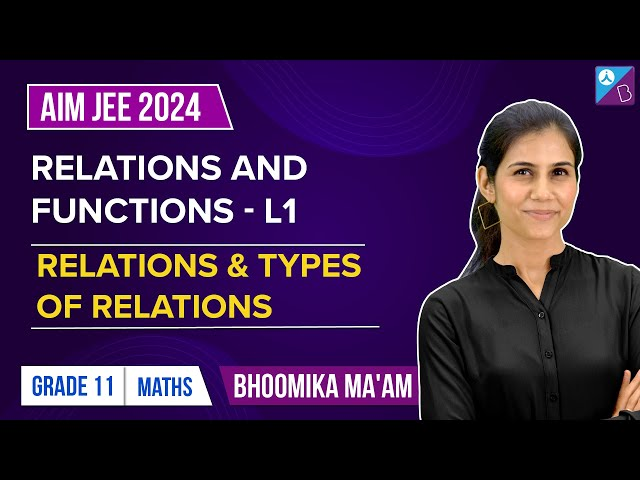### Functions – Introduction, Co-domain and RangeDownload BYJU’S – The Learning App and have fun learning with us.

Test your knowledge on Relations And Functions For Class 12